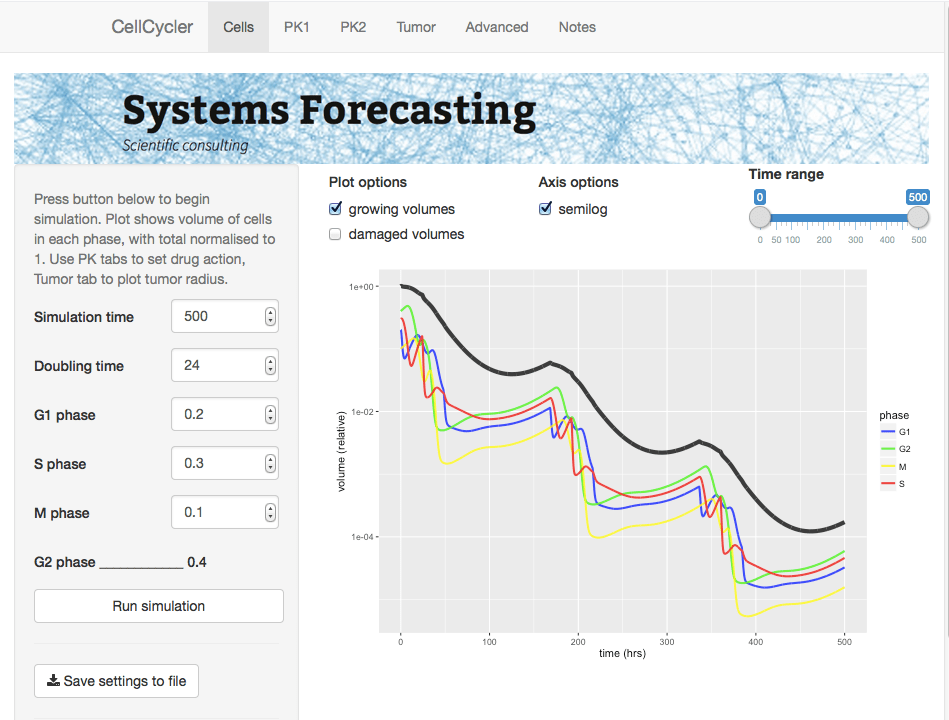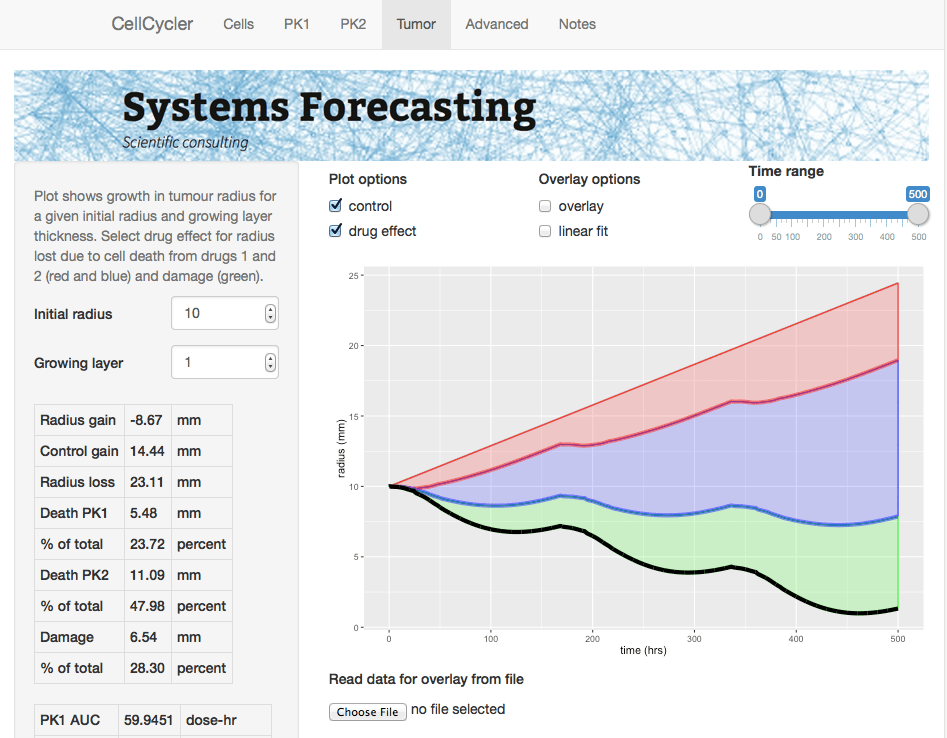# The CellCycler

Tumour modelling has been an active field of research for some decades, and a number of approaches have been taken, ranging from simple models of an idealised spherical tumour, to highly complex models which attempt to account for everything from cellular chemistry to mechanical stresses. Some models use ordinary differential equations, while others use an agent-based approach to track individual cells.

A disadvantage of the more complex models is that they involve a large number of parameters, which can only be roughly estimated from available data. If the aim is to predict, rather than to describe, then this leads to the problem of overfitting: the model is very flexible and can be tuned to fit available data, but is less useful for predicting for example the effect of a new drug.

Indeed, there is a rarely acknowledged tension in mathematical modelling between realism, in the sense of including lots of apparently relevant features, and predictive accuracy. When it comes to the latter, simple models often out-perform complex models. Yet in most areas there is a strong tendency for researchers to develop increasingly intricate models. The reason appears to have less to do with science, than with institutional effects. As one survey of business models notes (and these points would apply equally to cancer modelling) complex models are preferred in large part because: “(1) researchers are rewarded for publishing in highly ranked journals, which favor complexity; (2) forecasters can use complex methods to provide forecasts that support decision-makers’ plans; and (3) forecasters’ clients may be reassured by incomprehensibility.”

Being immune to all such pressures (this is just a blog post after all!) we decided to develop the CellCycler – a parsimonius “toy” model of a cancer tumour that attempts to capture the basic growth and drug-response dynamics using only a minimal number of parameters and assumptions.  The model uses circa 100 ordinary differential equations (ODEs) to simulate cells as they pass through the phases of the cell cycle; however the equations are simple and the model only uses parameters that can be observed or reasonably well approximated. It is available online as a Shiny app.Screenshot of the Cells page of the CellCycler. The plot shows how a cell population is affected by two different drugs.

The CellCycler model divides the cell cycle into a number of discrete compartments, and is therefore similar in spirit to other models that for example treat each phase G1, S, G2, and mitosis as a separate compartment, with damaged cells being shunted to their own compartment (see for example the model by Checkley et al. here). Each compartment has its own set of ordinary differential equations which govern how its volume changes with time due to growth, apoptosis, or damage from drugs. There are additional compartments for damaged cells, which may be repaired or lost to apoptosis. Drugs are simulated using standard PK models, along with a simple description of phase-dependent drug action on cells. For the tumour growth, we use a linear model, based like the Checkley et al. paper on the assumption of a thin growing layer (see also our post on The exponential growth effect).

Dividing the cell cycle into separate compartments has an interesting and useful side effect, which is that it introduces a degree of uncertainty into the calculation. For example, if a drug causes damage and delays progress in a particular phase, then that drug will tend to synchronize the cell population in that state. However there is an obvious difference between cells that are affected when they are at the start of the phase, and those that are already near the end of the phase. If the compartments are too large, that precise information about the state of cells is lost.

The only way to restore precision would be to use a very large number of compartments. But in reality, individual cells will not all have exactly the same doubling time. We therefore want to have a degree of uncertainty. And this can be controlled by adjusting the number of compartments.

This effect is illustrated by the figure below, which shows how a perturbation at time zero in one compartment tends to blur out over time, for models with 25, 50, and 100 compartments, and a doubling time of 24 hours. In each case a perturbation is made to compartment 1 at the beginning of the cell cycle (the magnitude is scaled to the number of compartments so the total size of the perturbation is the same in terms of total volume). For the case with 50 compartments, the curve after one 24 hours is closely approximated by a normal distribution with standard deviation of 3.4 hours or about 14 percent. In general, the standard deviation can be shown to be approximately equal to the doubling time divided by the square root of N.The solid lines show volume in compartment 1 following a perturbation to that compartment alone, after one cell doubling period of 24 hours. The cases shown are with N=25, 50, and 100 compartments. Dashed lines are the corresponding normal distributions.

A unique feature of the CellCycler is that it exploits this property as a way of adjusting the variability of doubling time in the cell population. The model can therefore provide a first-order approximation to the more complex heterogeneity that can be simulated using agent-based models. While we don’t usually have exact data on the spread of doubling times in the growing layer, the default level of 50 compartments gives what appears to be a reasonable degree of spread (about 14 percent). Using 25 compartments gives 20 percent, while using 100 compartments decreases this to 10 percent.

### Using the CellCycler

The starting point for the Shiny web application is the Cells page, which is used to model the dynamics of a growing cell population. The key parameters are the average cell doubling time, and the fraction spent in each phase. The number of model compartments can be adjusted in the Advanced page: note that, along with doubling time spread, the choice also affects both the simulation time (more compartments is slower), and the discretisation of the cell cycle. For example with 50 compartments the proportional phase times will be rounded off to the nearest 1/50=0.02.

The next pages, PK1 and PK2, are used to parameterise the PK models and drug effects. The program has a choice of standard PK models, with adjustable parameters such as Dose/Volume.  In addition the phase of action (choices are G1, S, G2, M, or all), and rates for death, damage, and repair can be adjusted. Finally, the Tumor page (shown below) uses the model simulation to generate a plot of tumor radius, given an initial radius and growing layer. Plots can be overlaid with experimental data.Screenshot of the Tumor page, showing tumor volume (black line) compared to control (grey). Cell death due to apoptosis by either drug (red and blue) and damage (green) are also shown.

We hope the CellCycler can be a useful tool for research or for exploring the dynamics of tumour growth. As mentioned above it is only a “toy” model of a tumour. However, all our models of complex organic systems – be they of a tumor, the economy, or the global climate system – are toys compared to the real things. And of course there is nothing to stop users from extending the model to incorporate additional effects. Though whether this will lead to improved predictive accuracy is another question.

Try the CellCycler web app here.

### References:

Stephen Checkley, Linda MacCallum, James Yates, Paul Jasper, Haobin Luo, John Tolsma, Claus Bendtsen. “Bridging the gap between in vitro and in vivo: Dose and schedule predictions for the ATR inhibitor AZD6738,” Scientific Reports.2015;5(3)13545.

Green, Kesten C. & Armstrong, J. Scott, 2015. “Simple versus complex forecasting: The evidence,” Journal of Business Research, Elsevier, vol. 68(8), pages 1678-1685.